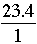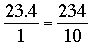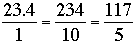SEARCH HOMEMath Central Quandaries & QueriesQuestion from Lisa, a parent: i am trying to turn a decimal into a fraction. my example is to turn 10.5 into a fraction at its lowest formHi Lisa,

Let me try 23.4, First I want to think of 23.4 as the fraction. To eliminate the decimal point and maintain an equivalent fraction I can multiply the numerator and denominator by to. thusThe numerator and denominator are both divisible by 2 so finally I getNow try this with 10.5,
PennyMath Central is supported by the University of Regina and The Pacific Institute for the Mathematical Sciences.